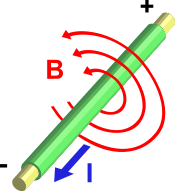# Electric Current Electric Charge & Electromagnetism

Very basic question which an electrical student or professional may be asked is what is electric current and electric charge. The electric current is actually the flow of electric charge from one point to other point of the conductor or wire or cable. In electric circuits this charge is often carried by moving electrons in a wire. It can also be carried by ions in an electrolyte, or by both ions and electrons such as in a plasma. I is used for symbolizing the current typically.

Ampere: Most common unit for measuring an electric current is the termed as ampere and denoted by A. One Ampere is the amount of electric charge across a surface at the rate of one coulomb per second.

Ammeter: A device which is used for measuring the flow of electric current is called an ammeter.

## Ohm’s law

Ohm’s law is used to demonstrate the relation between the electrical current and the voltage or potential difference. The law states that the flow of current through a conductor between two points is directly proportional to the potential difference across the two points. Introducing the constant of proportionality i.e R also called resistance of a conductor we come across the most famous and common equation of Ohm’s law:where I is the current through the conductor in units of amperes, V is the potential difference measured across the conductor in units of volts, and R is the resistance of the conductor in units of ohms. More specifically, Ohm’s law states that the R in this relation is constant, independent of the current which is different for each type of conductors depending upon the metal content etc.

## Electromagnetism

It is established that an electric current when flowing through a conductor produces magnetism effect and it is called the electromagnetism. If we wrap an insulated conductor on a cylindrical core in circular rounds and electric current is passed through the wire it becomes an electromagnet and once the current is off the magnetism affect is lost. The impact of electricity on a conductor is defined by Ampere’s law.

### Ampere’s law

According to Ampère’s law, an electric current produces a magnetic field. The magnetic field can be visualized as a pattern of circular field lines surrounding the wire that persists as long as there is current.Similarly magnetism can also produce electric currents. When a changing magnetic field is applied to a conductor, an Electromotive force (EMF) is produced, and when there is a suitable path, this causes current.

Electric current can be directly measured with a galvanometer, but this method involves breaking the electrical circuit, which is sometimes inconvenient and this is applied where we need to monitor the flow of current continuously. Typically in routine measurements current can be measured without breaking the circuit by detecting the magnetic field associated with the current i.e. from outside of the conductors/wires. Below is the list of devices used for measuring the electric current flow:

Source: 1

error: Content is protected !!